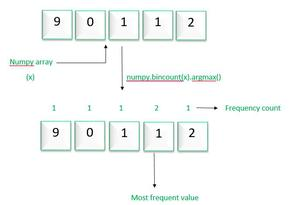# Find the most frequent value in a NumPy array

• Last Updated : 29 Aug, 2020

In this article, let’s discuss how to find the most frequent value in the NumPy array.

Steps to find the most frequency value in a NumPy array:

• Create a NumPy array.
• Apply bincount() method of NumPy to get the count of occurrences of each element in the array.
• The n, apply argmax() method to get the value having a maximum number of occurrences(frequency).Example 1:

## Python3

 `import` `numpy as np`` ` ` ` `# create array``x ``=` `np.array([``1``,``2``,``3``,``4``,``5``,``1``,``2``,``1``,``1``,``1``])``print``(``"Original array:"``)``print``(x)`` ` `print``(``"Most frequent value in the above array:"``)``print``(np.bincount(x).argmax())`

Output:

`1`

This code will generate a single output only, it will not work fine if the array contains more than one element having the maximum number of frequency.

Example 2: If the array has more than one element having maximum frequency

## Python3

 `import` `numpy as np`` ` ` ` `x ``=` `np.array([``1``, ``1``, ``1``, ``2``, ``3``, ``4``, ``2``, ``4``, ``3``, ``3``, ])``print``(``"Original array:"``)``print``(x)`` ` `print``(``"Most frequent value in above array"``)``y ``=` `np.bincount(x)``maximum ``=` `max``(y)`` ` `for` `i ``in` `range``(``len``(y)):``    ``if` `y[i] ``=``=` `maximum:``        ``print``(i, end``=``" "``)`

Output:

`1 3`

My Personal Notes arrow_drop_up